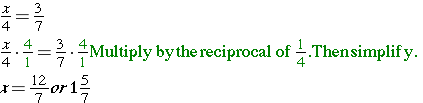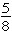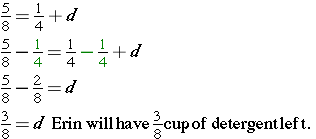# Solve Equations with Fractions

In this lesson, you will learn to solve equations containing fractions. The goal when you are solving equations with fractions is no different than when working with other types of numbers – isolate the variable on one side of the equation.

### Example 1

Solve equations by adding or subtracting:### Example 2

Solve equations by multiplying or dividing:

Fraction equations often call upon the Multiplicative Inverse Property

Multiplicative Inverse Property
Words Numbers Algebra
The product of a nonzero number and its reciprocal is one.## Investigate

Erin needscup of detergent for her laundry.  She hascup of detergent in the bottle. Write and solve an equation to find out how much detergent she will have left in the box.

Strategy

Summarize the problem, using only key words or phrases.

Erin hascup of detergent and needscup. How much will remain?

Next, translate the words into math. Use a variable to represent the remaining amount of detergent.

Total Detergent is the amount needed for laundry       and             the amount left=+ d

Solution(source)